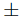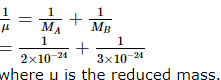Courses

# JEE(MAIN) Chemistry Mock Test - 7

## 30 Questions MCQ Test JEE Main Mock Test Series 2020 & Previous Year Papers | JEE(MAIN) Chemistry Mock Test - 7

Description
This mock test of JEE(MAIN) Chemistry Mock Test - 7 for JEE helps you for every JEE entrance exam. This contains 30 Multiple Choice Questions for JEE JEE(MAIN) Chemistry Mock Test - 7 (mcq) to study with solutions a complete question bank. The solved questions answers in this JEE(MAIN) Chemistry Mock Test - 7 quiz give you a good mix of easy questions and tough questions. JEE students definitely take this JEE(MAIN) Chemistry Mock Test - 7 exercise for a better result in the exam. You can find other JEE(MAIN) Chemistry Mock Test - 7 extra questions, long questions & short questions for JEE on EduRev as well by searching above.
QUESTION: 1

Solution:
QUESTION: 2

### Which of the following is hypnotic ?

Solution:
Solution :
Hypnotic or soporific drugs, commonly known as sleeping pills, are a class of psychoactive drugs whose primary function is to induce sleep and to be used in the treatment of insomnia, or surgical anesthesia.
QUESTION: 3

### Enzymes are made up of

Solution:
QUESTION: 4
The order of decrease in atomic radii for Be, Na and Mg is
Solution: As we move from left to right in a period, nuclear charge increases while the number of the shells remains the same. Due to this, the valence electrons are pulled little closer to the nucleus and this results in a decrease of the atomic radius. For example, when we move from Li to Be, atomic size decreases. On the other hand, the atomic radii of elements increase with increase in atomic numbers as we move from top to bottom in a group.This is due to the addition of new energy shells in each succeeding element. Thus, as we move from Li to Na, atomic radius increases and when go from Na to Mg, atomic radius decreases but not as much as in case of Be.
QUESTION: 5

Which of the following gives blood red colour with KCNS ?

Solution:
[B]
Fe+3 give blood red cloour with KCNS.
[Fe(CNS)2]+
x − 2 =  + 1
x = + 3
QUESTION: 6

Given that
(i) C + O₂ → CO₂ ; ∆Ho = − xkJ,
(ii) 2CO + O₂ → 2CO₂ ; ∆Ho = −ykJ .
The enthalpy of formation of carbon monoxide will be

Solution:
QUESTION: 7
The rate at which a substance reacts depends on its
Solution:
QUESTION: 8
[Co(NH₃)₄(NO₂)₂]Cl exhibits
Solution:
QUESTION: 9
Strong electrolytes are those which
Solution:
QUESTION: 10
Which of the following reagent can be used to convert benzene diazonium chloride into benzene ?
Solution:
QUESTION: 11

In the electrochemical cell Pt, H(g) 1 atm ∕ H+(I M) ∕ Cu2+ (I M) ∕ Cu(s) . Which one of the following statements is true?

Solution:
QUESTION: 12

pH of the solution at 250C is 2. If the pH is to be doubled then the hydronium ion concentration of the solution should be

Solution:
QUESTION: 13
The pH of 10 M HCl aqueous solution is
Solution:
QUESTION: 14

Given that Ka for acetic acid as 1.8x10⁻5 and Kb of NH₄OH as 1.8x10⁻5 at 25oC,predict the nature of aqueous solution of ammonium acetate

Solution:
QUESTION: 15
Highest pH (14) is shown by
Solution:
QUESTION: 16
Which of the following compound does not react with metallic sodium?
Solution:  (B) is methyl ether, presumably water free, and unreactive under normal conditions. Of course, at room temperature, it will be a gas.
QUESTION: 17
Which of the following polymer is formed when caprolactum is heated with the traces of water?
Solution:
QUESTION: 18
Ethane is obtained from ethylene by which of the following methods?
Solution:
QUESTION: 19
Which of the following is an example of body centred cube?
Solution:
QUESTION: 20
Which of the following metal oxides is antiferromagnetic in nature?
Solution:
QUESTION: 21

What among following is used to produce artificial rain ?

Solution:
QUESTION: 22
Number of moles of a solute per kilogram of a solvent is called
Solution:
QUESTION: 23
When an alkyne,RC ≡ CH, is treated with cuprous ion in an ammoniacal medium,one of the product is
Solution:
QUESTION: 24

If 20 ml of 0.4 N NaOH solution completely neutralises 40 ml of a dibasic acid, the molarity of the acid solution is

Solution:

NaOH Acid
N1V1 = N2V2
0.4 x 20 = N2 x 40
N2 = 0.4 x 20/40 = 0.2 N
Molarity = Normality x Eq wt of solute/Mol wt of solute
= Normality x 1/Basicity of acid
= 0.2 x 1/2 = 0.1 M

QUESTION: 25

For n = 5, total number of electrons will be ___

Solution:

Maximum number of electron in a shell
= 2n2
i.e., for n = 5
2 x 5 2 = 50 electrons.

QUESTION: 26

The quantum number of most energetic electron in Ne atom when it is in first excited state is__

Solution:

The electronic configuration of Ne atom is 1s2 2s2 2p6. In its first excitation state the electron from 2p level will unpair and shift to 3s where quantum number will be 3,0,0,1/2

QUESTION: 27

Since enthalpy of elements in their natural state is taken to the zero .The value of ∆Hf of compounds

Solution:
QUESTION: 28

If 2 x 10−24 gm and 3 x 10−24 gm are the masses of two atoms in a molecule, the reduced mass of the molecule is__

Solution:QUESTION: 29

Among the following metal carbonyls, the C --- O bond order is lowest in :

Solution:
QUESTION: 30

The empirical formula of an acid is CH₂O₂, the probable molecular formula of the simplest acid may be

Solution: